# Isolated Footing Design Example and Excel SheetReading time: 1 minute

Isolated footing design example with step by step procedure and isolated footing design excel sheet (spreadsheet) is also provided for easy and fast calculation. Learning design with examples is always the best method of learning. Step by step procedure for structural design of isolated footing is discussed below:

## Isolated Footing Design Example:

Let us consider an isolated footing for an RCC column of size 450mm x 450mm. Loads from this column to the foundation are: Vertical Load: 1000 kN Uniaxial Moment: 100 kNm The safe bearing capacity (SBC) of soil is 300 kN/m2. The grade of concrete to be used is M30 and grade of steel is Fe415.

### Step by Step Procedure of Isolated Footing Design:

Step -1: Determining size of footing: Loads on footing consists of load from column, self weight of footing and weight of soil above footing. For simplicity, self weight of footing and weight of soil on footing is considered as 10 to 15% of the vertical load. Load on column = 1000 kN Extra load at 10% of load due to self weight of soil = 1000 x 10% = 100kN Therefore, total load P = 1100 kN. Size of footing to be designed can be square, rectangular or circular in plan. Here we will consider square isolated footing. Therefore, length of footing (L) = Width of footing (B) Therefore area of footing required =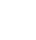= 1100/300 = 3.67 m2 Provide Length and width of footing = 2m Area of footing = 2 x 2 = 4m2 Now the pressure on isolated footing is calculated as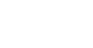When calculated, pmax = 325 kN/m2

pmin = 175 kN/m2

But pmax is greater than SBC of soil, so we need to revise the size of footing so that Pmax is below 300 kN/m2. Consider width and length of footing = L =B =2.25m Now, pmax = 250.21 kN/m2 (<300 kN/m2 -> OK) and pmin = 144.86 kN/m2 > 0 (OK) Hence, factored upward pressure of soil = pumax = 375.315 kN/m2 pumin = 217.29 kN/m2 Further, average pressure at the center of the footing is given by Pu,avg= 296.3 kN/m2 and, factored load, Pu= 1500 kN, factored uniaxial moment, Mu= 150 kN-m. Step 2: Two way shear Assume an uniform overall thickness of footing, D =500 mm Assuming 16 mm diameter bars for main steel, effective depth of footing ‘d’ is d = 500 – 50 – 8 = 452 mm The critical section for the two way shear or punching shear occurs at a distance of d/2 from the face of the column (Fig. 1), where a and b are the dimensions of the column.Fig 1: Critical section for Two Way Shear (Punching Shear) Hence, punching area of footing = (a + d)2 = (0.45 + 0.442)2 = 0.796 m2 where a = b = side of column Punching shear force = Factored load – (Factored average pressure x punching area of footing)

= 1500 – (296.3 x 0.0.796)

= 1264.245 kN

Perimeter along the critical section = 4 (a+d) = 4 (450+ 442) = 3568 mm Therefore, nominal shear stress in punching or punching shear stress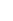is calculated as below: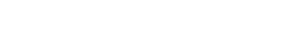= 1264.245 x 1000/(3568x442) = 0.802 N/mm2

Allowable shear stress =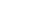where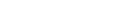= 1.369 N/mm2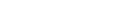=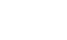=1

therefore, allowable shear stress = 1x1.369 = 1.369 N/mm2 Since the punching shear stress (0.802 N/mm2) is less than the allowable shear stress (1.369 N/mm2), the assumed thickness is sufficient to resist the punching shear force. Hence, the assumed thickness of footing D = 500 mm is sufficient. Please note, there is much difference between allowable and actual values of shear stress, so depth of footing can be revised and reduced. For our example, we will continue to use D = 500mm. Step 3: Design for flexure: The critical section for flexure occurs at the face of the column (Fig. 2).Fig. 2 Critical section for flexure

The projection of footing beyond the column face is treated as a cantilever slab subjected to factored upward pressure of soil. Factored maximum upward pressure of soil, pu,max= 375.315 kN/m2 Factored upward pressure of soil at critical section, pu= 312.1 kN/m2 Projection of footing beyond the column face, l = (2250 – 450)/2 = 900 mm Bending moment at the critical section in the footing is given by: Mu = Total force X Distance from the critical section Considering uniform soil pressure of 375.315, Mu = 180 kN/m2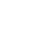0.92

from SP 16, percentage of reinforcement can be found for M30 concrete, fe415 steel for abovept = 0.265%

Ast = pt x bxd

considering 1m wide footing, Ast required = 1171.1 mm2/ m width Provide 16 dia bar @ 140mm c/c Repeat this exercise for other direction as well. Since, uniform base pressure is assumed, and it is a square footing, Mu and Ast for other direction will be same. Step 4: Check for One-Way Shear: The critical section for one way shear occurs at a distance of ‘d’ from the face of the column. Factored maximum upward pressure of soil, pu,max= 375.315 kN/m2 Factored upward pressure of soil at critical section, pu= 375.315 kN/m2 For the cantilever slab, total Shear Force along critical section considering the entire width B is

Vu = Total Force X (l - d) X B

= 375.315 X (0.9 - 0.442) X 2 = 343.8 kN

Nominal shear stress = Vu/(Bxd) = 0.346 N/mm2

For, pt = 0.265, and M30, allowable shear force from Table - 19, IS456 is greater than 0.346 N/mm2 Therefore, the foundation is safe in one-way shear. Step 5: Check for development length Sufficient development length should be available for the reinforcement from the critical section. Here, the critical section considered for Ld is that of flexure. The development length for 16 mm diameter bars is given by Ld= 47xdiameter of bar = 47 x 16 = 752 mm. Providing 60 mm side cover, the total length available from the critical section is 0.5x( L - a) - 60 = 0.5x(2250 - 450) - 60 = 840 > Ld, Hence O.K. Download Isolated Footing Design Excel Calculation Read More: What is Punching Shear? Punching Shear in Slabs and Foundations Combined Footing Design with Example and Types of Combined Footing